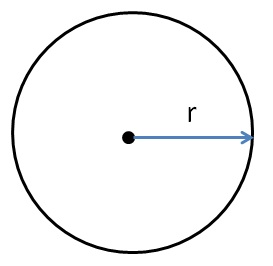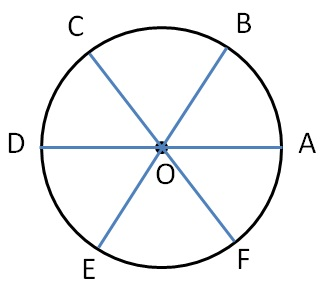Circles

Chapter 4 Class 6 Basic Geometrical Ideas
Concept wiseDistance of center from any other point on the circle is the radius.Here,

Radius = (OA)  = (OB)  = (OC)  = (OD)  = (OE)  = (OF)

Learn in your speed, with individual attention - Teachoo Maths 1-on-1 Class